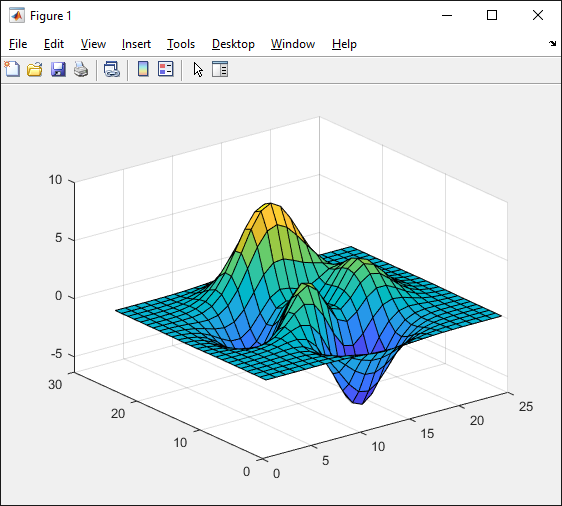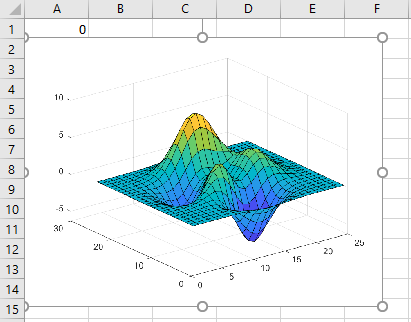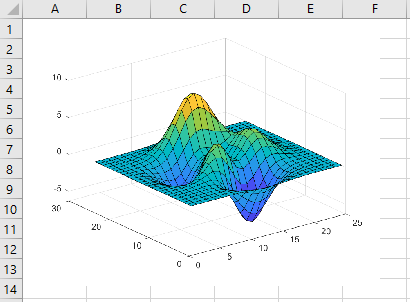# MLGetFigure

Import current MATLAB figure into Microsoft Excel worksheet

## Syntax

``= MLGetFigure(width, height)``
``MLGetFigure width, height``
``out = MLGetFigure(width,height)``

## Description

example

````= MLGetFigure(width, height)` imports the current MATLAB® figure into an Excel® worksheet, placing the top-left corner of the figure in the current worksheet cell. Specify the normalized width and height of the figure in Excel. Use this syntax when working directly in a worksheet.```

example

````MLGetFigure width, height` imports the current MATLAB figure into an Excel worksheet, placing the top-left corner of the figure in the current worksheet cell. Use this syntax in a VBA macro.```
````out = MLGetFigure(width,height)` lets you find errors when executing `MLGetFigure` in a VBA macro. If `MLGetFigure` fails, then `out` is a string containing an error code. Otherwise, `out` is `0`.```

## Examples

collapse all

After you create a figure in MATLAB, import the figure into an Excel worksheet.

Note

If you use Excel 2007 or 2010, the width and height of the imported figure will be a quarter of the size of the original figure.

Create a wireframe mesh in MATLAB using the `peaks` and `surf` functions. The figure window displays a wireframe mesh.

```z = peaks(25); surf(z)```Open Excel and make sure cell `A1` is selected in the worksheet. Import the current figure into the worksheet using the `MLGetFigure` function. Enter this text in the cell and press Enter.

`= MLGetFigure(.8, .8)`

The `MLGetFigure` function imports the current figure into the worksheet, placing the top-left corner of the figure in the selected cell.After you create a figure in MATLAB, import the figure into an Excel worksheet using a VBA macro.

Create a wireframe mesh in MATLAB using the `peaks` and `surf` functions. The figure window displays a wireframe mesh.

```z = peaks(25); surf(z)```On the Developer tab in Excel, click in the Code group. The Visual Basic® Editor window opens.

Select Insert > Module to insert a new module. In the Module1 window, enter this VBA code containing a macro named `MyFigure`.

```Sub MyFigure() MLGetFigure 0.8, 0.8 MatlabRequest End Sub```

The `MyFigure` macro uses the `MLGetFigure` function to import the current figure into the worksheet. `MatlabRequest` initializes internal Spreadsheet Link™ variables and enables `MLGetFigure` to function in a subroutine.

For details about working with modules, see Excel Help.

Select cell `A1` for the position of the figure. Run the macro by clicking button on the VBA toolbar. For details about running macros, see Excel Help.

The `MLGetFigure` function imports the current figure into the worksheet, placing the top-left corner of the figure in the selected cell.## Input Arguments

collapse all

Width (in normalized units) of the MATLAB figure when imported into an Excel worksheet, specified as a real number.

Example: `0.5`

Height (in normalized units) of the MATLAB figure when imported into an Excel worksheet, specified as a real number.

Example: `0.5`

## Tips

• If you use Microsoft® Excel 2007 or 2010, `MLGetFigure` scales the imported figure by the product of `width` and `height` along both dimensions.

• If worksheet calculation mode is automatic, the software executes `MLGetFigure` when you enter the formula in a cell. If worksheet calculation mode is manual, enter the `MLGetFigure` function in a cell, then press `F9` to execute it. Note that pressing `F9` can also execute other worksheet functions and generate unpredictable results.

• If you use `MLGetFigure` in a macro subroutine, enter `MatlabRequest` on the line after `MLGetFigure`. The execution of `MatlabRequest` initializes internal Spreadsheet Link variables and enables `MLGetFigure` to function in a subroutine. Do not include `MatlabRequest` in a macro function unless the function is called from a subroutine.

• To work with VBA code in Excel with Spreadsheet Link, you must enable Spreadsheet Link as a reference in the Microsoft Visual Basic Editor. For details, see Installation.